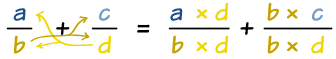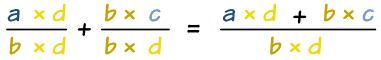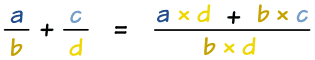# Common Denominator

This is the easiest method we know to add or subtract fractions!

## What is a Denominator?The denominator is the bottom number in a fraction.

It shows how many equal parts the item is divided into.## What is a Common Denominator?

When the denominators of two or more fractions are the same, they are Common Denominators.

## Why is it Important?

Before we can add or subtract fractions, the fractions need to have a common denominator

Iin other words the denominators must be the same.

## Making The Denominators the Same

To make the denominators the same we can:

Multiply top and bottom of each fraction by the denominator of the other.

Like in this example (press play button):

This always works, but we often need to simplify the fraction afterwards, as in this example (press play button):

We simplified the fraction 2032 to 1016 , then to 58 by dividing the top and bottom by 2 each time, and that is as simple as it can get!

## What Did We Do?

1. We multiplied each fraction by the denominator of the other. Let's use letters instead of numbers to show it:2. And because they now have the same denominator, we can add them:### In One Step!

We could do those two things in one step like this:Which we can use like this:

### Example: What is 23 + 45 ?

23 + 45 = 2×5 + 3×43×5 = 10 + 1215 = 2215

(Note: a was 2, b was 3, c was 4 and d was 5.)

That is how the experts do it!

### Footnote: Least Common Denominator

You can also try the Least Common Denominator method.